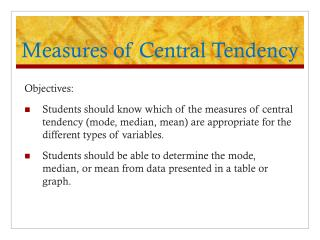# Measures of Central Tendency - PowerPoint PPT PresentationDownload PresentationMeasures of Central Tendency

Measures of Central TendencyDownload Presentation## Measures of Central Tendency

- - - - - - - - - - - - - - - - - - - - - - - - - - - E N D - - - - - - - - - - - - - - - - - - - - - - - - - - -
##### Presentation Transcript

1. Measures of Central Tendency Objectives: • Students should know which of the measures of central tendency (mode, median, mean) are appropriate for the different types of variables. • Students should be able to determine the mode, median, or mean from data presented in a table or graph.

2. Measures of Central Tendency Single numerical descriptive measures that locate the center, or “typical” value of a distribution of data. The three most widely used measures of central tendency are: mode, median, mean

3. Mode The most frequently occurring value (or category) in a data set. A data set is bimodal if it contains two values that are tied for the highest frequency of occurrence. A data set can also be without a mode, if no value occurs more often than any other. In the data set {20, 30, 30, 30, 35, 39, 46, 58, 59} the mode is 30, because it occurs more often than any other value.

4. Mode The mode can be obtained for any type of variable, whether nominal, ordinal, or quantitative (continuous or discrete). However, the mode is the only measure of central tendency that can used for nominal data.

5. Chief complaints of a sample of patients presenting to the Emergency Department (n=614) in November, 2008 Example: the mode for a nominal variable The mode is “Chest Pain”

6. Median The middle value in a set of data. Exactly half of the values lie below it, and half lie above it. Determine by: First arrange the data values in order from lowest to highest. For a data set with an odd number of observations, the median is the middle value. For a data set with an even number of observations, the median is the average of the two middle values.

7. Median In the data set on a previous slide, the values were: {20, 30, 30, 30, 35, 39, 46, 58, 59} There are 9 values- the median is the 5th value: {20, 30, 30, 30, 35, 39, 46, 58, 59} If we add a 10th value, the median is the average of the 5th and 6th values: {20, 30, 30, 30, 35, 39, 46, 58, 59, 60} (35+39)/2 = 37

8. Median The median can be used as a measure of central tendency for ordinal or quantitative variables. Example: the median for an ordinal variable: There are 400 observations: the median is the 200th-201st observation, which falls in the “Very important” category

9. Mean The average: the sum of the values in a set of observations, divided by the total number of observations. General formula: X = the mean X = the sum of the observations N = the total number of observations The mean can be used as a measure of central tendency forquantitative/numericaldata only.

10. Example n= 30: 0, 0, 1, 1, 2, 2, 2, 3, 3, 3 4, 4, 4, 4, 4, 4, 4, 4, 4, 4 5, 5, 6, 6, 6, 7, 7, 8, 9, 9 Mode: most frequently occurring value = 4 Median: exact middle value = average of 15th and 16th value = (4 + 4) / 2 = 8 / 2 = 4 Mean: sum of observations /n = 125 / 30 = 4.2

11. Which Measure is Best? The most important factor to consideris: the level of measurement or type of variable Nominal variables: the mode is the only appropriate measure of central tendency. Ordinal variables: both the mode and median are used. Quantitative variables: mode, median, and mean can all be used. Mean is usually the measure of choice because it is a unique value, it uses all values in the data set, and it can be used in subsequent analyses. However, the mean is subject to extreme (high or low) values (outliers). If the data set contains extreme values, calculate both median & mean.